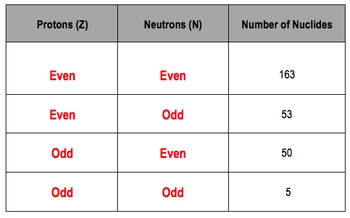Start typing, then use the up and down arrows to select an option from the list.## General Chemistry

Learn the toughest concepts covered in Chemistry with step-by-step video tutorials and practice problems by world-class tutors

21. Nuclear Chemistry

Magic numbers are an established number of nucleons (either neutrons or protons) that make a nucleus especially stable.

The Magic Numbers

Whereas the Octet Rule relates stability to the number of valence electrons, the Magic Numbers relate the stability of the nucleus to the ratio of neutrons to protons.

1
concept

## Magic Numbers4m
Play a video:2
concept

## Magic Numbers2m
Play a video:
So we're gonna continue and saying in terms of electrons and stability, remember, the noble gasses are the group on the periodic table that all the elements try to become. Why? Because they have the ideal number of outer electrons, their outer shells air completely filled. Now, here, if we look at a normal periodic table, these here these atomic numbers, when an element is neutral that represent the number of protons but more importantly, also the number of electrons. So here these are the ideal numbers for the number of electrons you want to be. A stable is possible. Okay, so those are the numbers will write in. So we have here too. We have 10. We have 18 here. We had 36. So all we're doing is we're filling in the periodic table with the atomic numbers of each of these elements. We had 54 for Zen on 86 for Radan, and then we had 1 18 as the last one. Now, just as elements with the ideal number of electrons depict unusually high stability, so do elements with the ideal number of nuclear. Iran's nuclear is just referring to number of protons and neutrons these ideal numbers for nuclear ions are referred to as our magic numbers. So basically, if you have a new isotope that has any one of these magic numbers, it will represent ah, stable isotope for that element. Now, we're gonna say, in terms of nuclear arms and civility, the magic numbers include So for protons, they include two, 8 20 28 82 then 1 14. So these air number that you should commit to memory, Then we're going to say here that for neutrons, some of them are the same in the very beginning, so we still have to 8 20 50 and 82. So that's what they have in common with one another these initial numbers. But then we have a difference for the last two word. This is 1 26 and then this is 1 84. Okay, so those would be the magic numbers for our number of neutrons. Now, based on this, let's see if you guys can answer the following example that's on the bottom of the page. Don't worry. If you get stuck, just come back. Take a look at how I approach that question to find the correct answer
3
example

## Magic Numbers2m
Play a video: